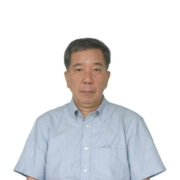6-6 Reduction of order IIIntroduction to Ordinary Differential Equations
201 個評分Korea Advanced Institute of Science and Technology(KAIST)

Introduction to Ordinary Differential Equations

201 個評分

Linear Second order equations 2

與講師見面

•Kwon, Kil Hyun
Professor
Department of Mathematical Sciences